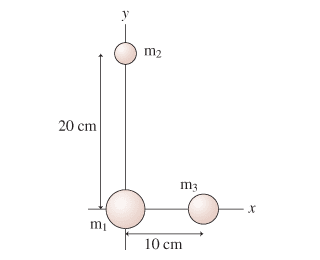# Gravitational force between 3 masses

• HaLAA

## Homework Statement

What is the magnitude of the net gravitational force on the m2=5kg mass? Assume m1=25kg and m3=15kg.
https://session.masteringphysics.com/problemAsset/1383524/6/13_P28.png

F=GMm/r^2

## The Attempt at a Solution

First I find the angle between m1 and m2 which is arctan(10/20)=27
Find the force m3 on m2 which is

F23=Gm2m3/0.22^2(cos27i-sin27j)=8.91*10^-9Ni-4.54*10^-9Nj
There also a force acting m2 on m1 which is
F21=-Gm1m2/0.2^2=-2.09*10^-7Nj

the net force, I do Fnet=sqrt((8.91*10^-9Ni)^2+(-2.09*10^-7Nj-4.54*10^-9Nj)^2)=2.137*10^-7N

However, the answer I got is incorrect.

can anyone tell me where I did wrong?

Thanks![/B]

the angle between m1 and m2 which is arctan(10/20)=27
Please clarify exactly what angle you believe you have calculated, then check whether that is the angle you use later in the trig expressions.

Please clarify exactly what angle you believe you have calculated, then check whether that is the angle you use later in the trig expressions.the angle is between line m1m2 and line m2m3 (if we connect m2and m3)

the angle is between line m1m2 and line m2m3 (if we connect m2and m3)
Then double check how that relates to your i and j vectors here (j is vertical in the diagram, yes?):
F23=Gm2m3/0.22^2(cos27i-sin27j)

Then double check how that relates to your i and j vectors here (j is vertical in the diagram, yes?):
F23 should be Gm2m3/0.22^2(cos27j-sin27i),
I get 2.0*10^-7N, but it still incorrect

F23 should be Gm2m3/0.22^2(cos27j-sin27i),
I get 2.0*10^-7N, but it still incorrect
Certainly that's not what I get.

Certainly that's not what I get.
F21=-Gm1m2/0.2^2=-2.09*10^-7Nj
F23=Gm2m3/0.22^2(sin27i-cos27j)=4.6*10^-9Ni-8.9*10^-9Nj
There also a force acting m2 on m1 which is
F21=-Gm1m2/0.2^2=-2.09*10^-7Nj

the net force, I do Fnet=sqrt((4.6*10^-9Ni)^2+(-2.09*10^-7Nj-8.9*10^-9Nj)^2)=2.0*10^-7N

F21=-Gm1m2/0.2^2=-2.09*10^-7Nj
F23=Gm2m3/0.22^2(sin27i-cos27j)=4.6*10^-9Ni-8.9*10^-9Nj
There also a force acting m2 on m1 which is
F21=-Gm1m2/0.2^2=-2.09*10^-7Nj

the net force, I do Fnet=sqrt((4.6*10^-9Ni)^2+(-2.09*10^-7Nj-8.9*10^-9Nj)^2)=2.0*10^-7N
That's no more detail than you already provided.
Here's a guess: what do you get for e.g. sin(27)? Remember to discriminate degrees from radians.

That's no more detail than you already provided.
Here's a guess: what do you get for e.g. sin(27)? Remember to discriminate degrees from radians.

First, because m2 is above m1 and m3 and there is a angle between them which is 27degree.

the force on F23 = Gm2m3/0.22^2(sin(27)i-cos(27)j)=4.6*10^-9Ni(pointing to the right)-8.9*10^-9Nj(pointing down)

there is a force F12 but is -F21 because the force is from m2 to m1

F21=-Gm1m2/0.2^2=-2.09*10^-7Nj

to get the net force, I need to use the Pythagorean theorem, i+i and j+j to make sure I add up the right directional unit vector
Fnet=sqrt((4.6*10^-9Ni)^2+(-2.09*10^-7Nj-8.9*10^-9Nj)^2)=2.0*10^-7N

That's adding description, not arithmetic detail. Break this one down:
Gm2m3/0.22^2(sin(27)i)=4.6*10^-9Ni(pointing to the right)
I get a different value, nearly 10 times as much.

That's adding description, not arithmetic detail. Break this one down:

I get a different value, nearly 10 times as much.
it is 4.67*10^-8

it is 4.67*10^-8
Much closer, but you are getting some rounding errors by using the angle 27 degrees instead of something more accurate.
(It isn't necessary to calculate the angle at all - just express the cos and sin values in terms of the given distances.)
Still not what I get for the final answer though. And I don't agree with this:
-8.9*10^-9Nj(pointing down)

Much closer, but you are getting some rounding errors by using the angle 27 degrees instead of something more accurate.
(It isn't necessary to calculate the angle at all - just express the cos and sin values in terms of the given distances.)
Still not what I get for the final answer though. And I don't agree with this:
I plug these number in wolfram get 6.67*10^-11*15*5/0.22^2(0.1/0.22)=4.698*10^-8,
I want to know why -8.9*10^-9Nj(pointing down) isn't pointing down.

6.67*10^-11*15*5/0.22^2(0.1/0.22),
The 0.1 is wrong. But even with 0.1 I don't get 8.9*10^-9. I plugged the above into a spreadsheet as 6.67*10^-11*15*5/0.22^2*(0.1/0.22) and got 4.7*10^-8.
why -8.9*10^-9Nj(pointing down) isn't pointing down.
It's pointing down.. it's the number I disagree with.

The 0.1 is wrong. But even with 0.1 I don't get 8.9*10^-9. I plugged the above into a spreadsheet as 6.67*10^-11*15*5/0.22^2*(0.1/0.22) and got 4.7*10^-8.

It's pointing down.. it's the number I disagree with.
now I think I get all right number, Fnet=sqrt((4.698*10^-8Ni)^2+(-2.09*10^-7Nj-9.369*10^-8Nj)^2)=3.07*10^-7N

now I think I get all right number, Fnet=sqrt((4.698*10^-8Ni)^2+(-2.09*10^-7Nj-9.369*10^-8Nj)^2)=3.07*10^-7N
I get 3.01*10^-7. You lose accuracy by calculating intermediate results and plugging them back in later.

You lose accuracy by calculating intermediate results and plugging them back in later.

More precisely, you lose accuracy by calculating intermediate results, rounding them off, and then plugging the rounded values back in later.

Ideally, you should keep the intermediate results in your calculator, using memory registers if necessary. Round off only at the end, when you have your final result. If you must write down intermediate results because you're using a stone-age calculator, keep 2 or 3 extra significant figures.